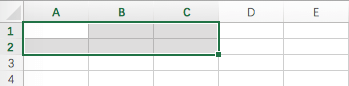# 用 Python 读写 Excel 表格

2019/04/10 10:10

Python 可以读写 Excel 表格吗？

openpyxl 的设计非常漂亮 ，你一定会喜欢它！不信请往下看：

# 工作簿

``````>>> from openpyxl import Workbook
>>> wb = Workbook()
``````

``````>>> ws = wb.active
``````

``````>>> ws1 = wb.create_sheet('Mysheet')
``````

``````>>> ws1 = wb.create_sheet('Mysheet', 0)
``````

``````>>> ws.title = 'New Title'
``````

``````>>> ws.sheet_properties.tabColor = "1072BA"
``````

``````>>> ws3 = wb["New Title"]
``````

``````>>> print(wb.sheetnames)
['Sheet2', 'New Title', 'Sheet1']
``````

``````>>> for sheet in wb:
...     print(sheet.title)
``````

``````>>> source = wb.active
>>> target = wb.copy_worksheet(source)
``````

## 从文件加载

``````>>> from openpyxl import load_workbook
>>> print(wb2.sheetnames)
['Sheet2', 'New Title', 'Sheet1']
``````

# 数据处理

## 单个单元格

``````>>> cell = ws['A4']
``````

``````>>> ws['A4'] = 10
``````

``````>>> cell = ws.cell(row=4, column=2, value=10)
``````

## 多单元格

``````>>> cell_range = ws['A1':'C2']
````````````# 取出 C 列
>>> colC = ws['C']

# 取出 C 至 D 列
>>> col_range = ws['C:D']

# 取出第 10 行
>>> row10 = ws

# 取出第 5 至 10 行
>>> row_range = ws[5:10]
``````

``````# 从第 1 行开始遍历，直到第 2 行，每行最多返回 3 列
>>> for row in ws.iter_rows(min_row=1, max_row=2, max_col=3):
...    for cell in row:
...        print(cell)
<Cell Sheet1.A1>
<Cell Sheet1.B1>
<Cell Sheet1.C1>
<Cell Sheet1.A2>
<Cell Sheet1.B2>
<Cell Sheet1.C2>
``````

``````>>> ws = wb.active
>>> ws['C9'] = 'hello world'
>>> tuple(ws.rows)
((<Cell Sheet.A1>, <Cell Sheet.B1>, <Cell Sheet.C1>),
(<Cell Sheet.A2>, <Cell Sheet.B2>, <Cell Sheet.C2>),
(<Cell Sheet.A3>, <Cell Sheet.B3>, <Cell Sheet.C3>),
(<Cell Sheet.A4>, <Cell Sheet.B4>, <Cell Sheet.C4>),
(<Cell Sheet.A5>, <Cell Sheet.B5>, <Cell Sheet.C5>),
(<Cell Sheet.A6>, <Cell Sheet.B6>, <Cell Sheet.C6>),
(<Cell Sheet.A7>, <Cell Sheet.B7>, <Cell Sheet.C7>),
(<Cell Sheet.A8>, <Cell Sheet.B8>, <Cell Sheet.C8>),
(<Cell Sheet.A9>, <Cell Sheet.B9>, <Cell Sheet.C9>))
``````

``````>>> tuple(ws.columns)
((<Cell Sheet.A1>,
<Cell Sheet.A2>,
<Cell Sheet.A3>,
<Cell Sheet.A4>,
<Cell Sheet.A5>,
<Cell Sheet.A6>,
...
<Cell Sheet.B7>,
<Cell Sheet.B8>,
<Cell Sheet.B9>),
(<Cell Sheet.C1>,
<Cell Sheet.C2>,
<Cell Sheet.C3>,
<Cell Sheet.C4>,
<Cell Sheet.C5>,
<Cell Sheet.C6>,
<Cell Sheet.C7>,
<Cell Sheet.C8>,
<Cell Sheet.C9>))
``````

# 数据存储

Excel 表格通过单元格存储数据，直接赋值即可：

``````>>> cell.value = 'hello, world'
>>> print(cell.value)
'hello, world'

>>> cell2.value = 3.14
>>> print(cell2.value)
3.14
``````

``````>>> wb = Workbook(guess_types=True)
``````

``````>>> cell.value = '31.50'
>>> print(cell.value)
31.5

>>> cell2.value = '12%'
>>> print(cell2.value)
0.12
``````

``````>>> import datetime
>>> cell.value = datetime.datetime.now()
>>> print cell.value
datetime.datetime(2010, 9, 10, 22, 25, 18)
``````

## 保存至文件

``````>>> wb = Workbook()
>>> wb.save('balances.xlsx')
``````

``````>>> wb = load_workbook('document.xlsx')
>>> wb.template = True
>>> wb.save('document_template.xltx')
``````

``````>>> wb = load_workbook('document_template.xltx')
>>> wb.template = False
>>> wb.save('document.xlsx', as_template=False)
``````

## 保存至流

FlaskDjangoWeb 应用，可能需要将文件保存到流( stream )。 借助一个临时文件( NamedTemporaryFile )可以轻松实现：

``````>>> from tempfile import NamedTemporaryFile
>>> from openpyxl import Workbook
>>> wb = Workbook()

# 先保存到临时文件，再将文件内容读出
>>> with NamedTemporaryFile() as tmp:
...     wb.save(tmp.name)
...     tmp.seek(0)
``````

# 附录

0
0 收藏

### 作者的其它热门文章0 评论
0 收藏
0